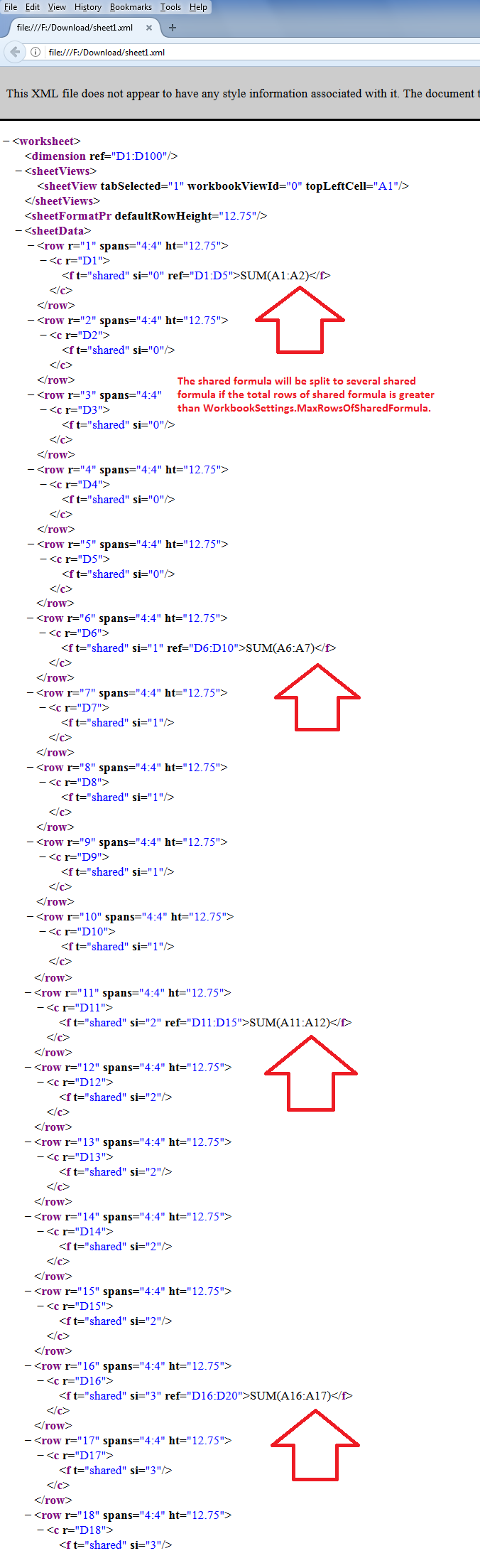# Specify Maximum Rows of Shared Formula

Contents
[ ]

## Possible Usage Scenarios

The default maximum rows of the shared formula are 64. It could be any number e.g. it could be 1000. The performance of shared formula changes with a different number of rows. Therefore, Aspose.Cells provides the  Workbook.Settings.MaxRowsOfSharedFormula  property that can be used to specify the maximum rows of the shared formula. The shared formula will be split to several shared formulae if the total rows of the shared formula are greater than it as shown in the following screenshot.## Specify Maximum Rows of Shared Formula

The following sample code explains the usage of the Workbook.Settings.MaxRowsOfSharedFormula property. It sets the maximum rows of the shared formula to 5 and adds the shared formula in cell D1 for 100 rows and saves to output Excel file. If you extract the contents of output Excel file and check the sheet1.xml, you will see the shared formula splits after every 5 rows as highlighted in the above screenshot.